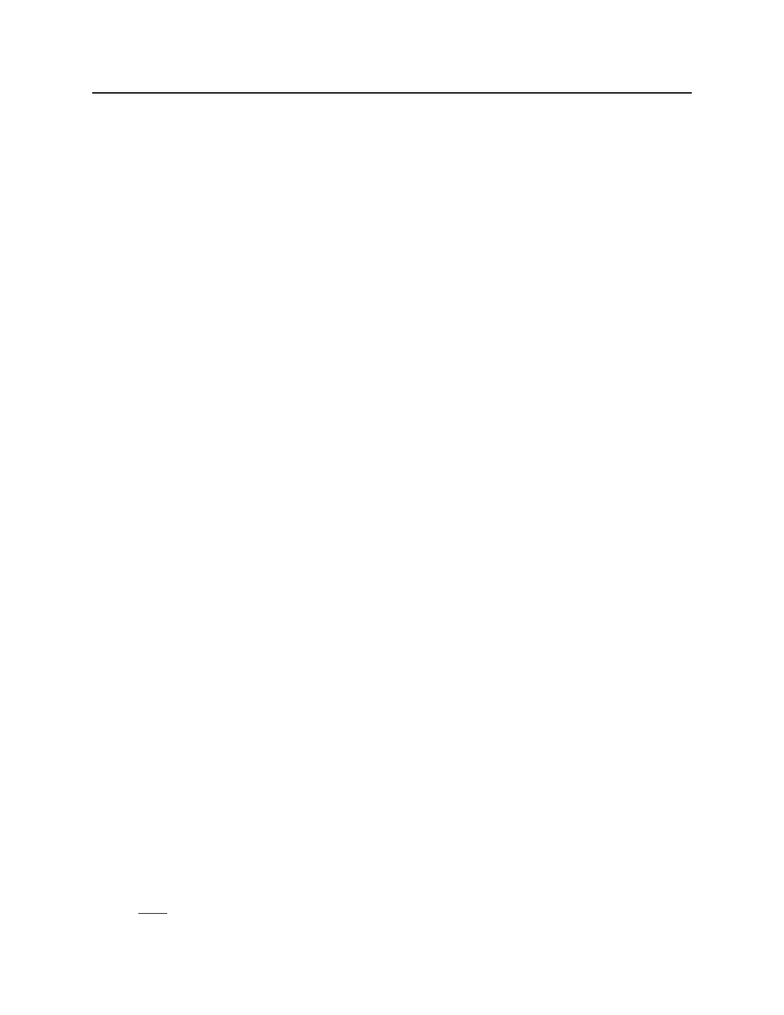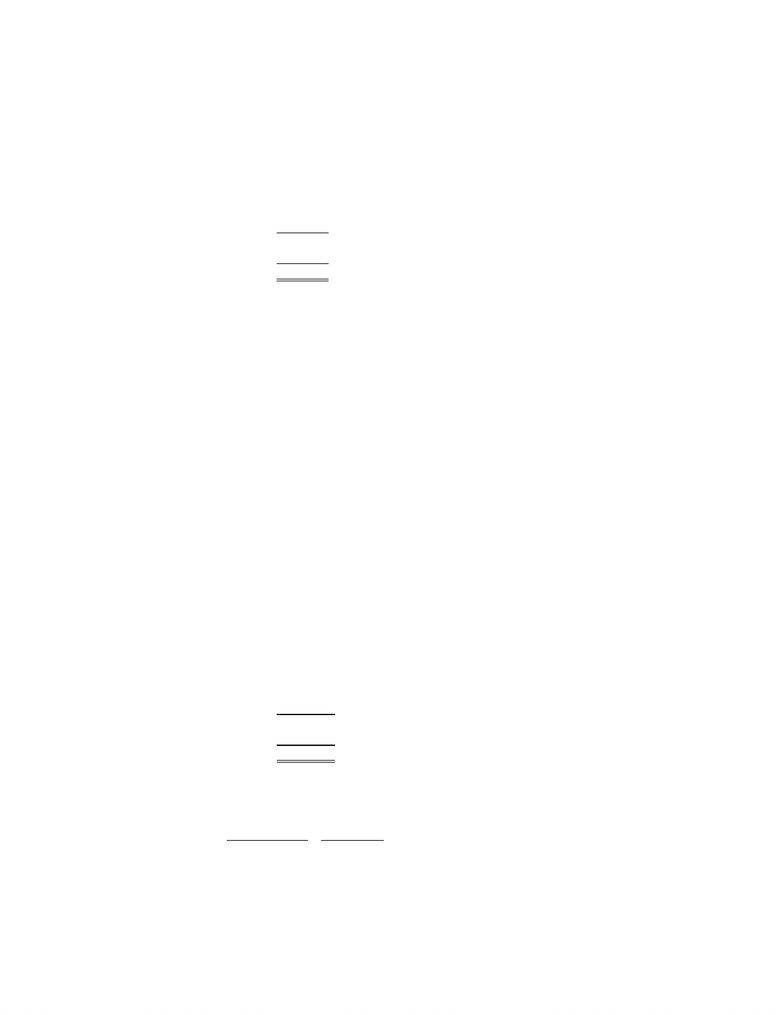Study Guides (390,000)
CA (150,000)
Ryerson (10,000)
Finance (300)
FIN 300 (100)

# FIN 300 Study Guide - Tax Shield, Cash Flow, Net Income

Department
Finance
Course Code
FIN 300
Professor
Scott Anderson

This preview shows pages 1-3. to view the full 18 pages of the document.CHAPTER 10
Making Capital Investment Decisions
Answers to Concepts Review and Critical Thinking Questions
1. An opportunity cost is the most valuable alternative that is foregone if a particular project is undertaken. The
relevant opportunity cost is what the asset or input is actually worth today, not, for example, what it cost to
acquire.
2. It’s probably only a mild over-simplification. Current liabilities will all be paid presumably. The cash portion
of current assets will be retrieved. Some receivables won’t be collected, and some inventory will not be sold,
of course. Counterbalancing these losses is the fact that inventory sold above cost (and not replaced at the end
of the project’s life) acts to increase working capital. These effects tend to offset.
3. The EAC approach is appropriate when comparing mutually exclusive projects with different lives that will be
replaced when they wear out. This type of analysis is necessary so that the projects have a common life span
over which they can be compared; in effect, each project is assumed to exist over an infinite horizon of N-year
repeating projects. Assuming that this type of analysis is valid implies that the project cash flows remain the
same forever, thus ignoring the possible effects of, among other things: (1) inflation, (2) changing economic
conditions, (3) the increasing unreliability of cash flow estimates that occur far into the future, and (4) the
possible effects of future technology improvement that could alter the project cash flows.
4. Depreciation is a non-cash expense, but it is tax-deductible on the income statement. Thus depreciation causes
taxes paid, an actual cash outflow, to be reduced by an amount equal to the depreciation tax shield tcD. A
reduction in taxes that would otherwise be paid is the same thing as a cash inflow, so the effects of the
depreciation tax shield must be included to get the total incremental aftertax cash flows.
5. There are two particularly important considerations. The first is erosion. Will the essentialized book simply
displace copies of the existing book that would have otherwise been sold? This is of special concern given the
lower price. The second consideration is competition. Will other publishers step in and produce such a
product? If so, then any erosion is much less relevant. A particular concern to book publishers (and producers
of a variety of other product types) is that the publisher only makes money from the sale of new books. Thus, it
is important to examine whether the new book would displace sales of used books (good from the publisher’s
perspective) or new books (not good). The concern arises any time there is an active market for used product.
6. This market was heating up rapidly, and a number of other competitors were planning on entering. Any
erosion of existing services would be offset by an overall increase in market demand.
7. Air Canada should have realized that abnormally large profits would dwindle as more supply of services came
into the market and competition became more intense.
Solutions to Questions and Problems
NOTE: All end of chapter problems were solved using a spreadsheet. Many problems require multiple steps. Due to
space and readability constraints, when these intermediate steps are included in this solutions manual, rounding
may appear to have occurred. However, the final answer for each problem is found without rounding during any
step in the problem.
Basic
97
www.notesolution.com

Only pages 1-3 are available for preview. Some parts have been intentionally blurred.1. The \$5 million acquisition cost of the land six years ago is a sunk cost. The \$5.4 million current aftertax value
of the land is an opportunity cost if the land is used rather than sold off. The \$10.4 million cash outlay and
\$650,000 grading expenses are the initial fixed asset investments needed to get the project going. Therefore,
the proper year zero cash flow to use in evaluating this project is
\$5,400,000 + 10,400,000 + 650,000 = \$16,450,000
2. Sales due solely to the new product line are:
21,000(\$12,000) = \$252,000,000
Increased sales of the motor home line occur because of the new product line introduction; thus:
5,000(\$45,000) = \$225,000,000
in new sales is relevant. Erosion of luxury motor coach sales is also due to the new mid-size campers; thus:
1,300(\$85,000) = \$110,500,000 loss in sales
is relevant. The net sales figure to use in evaluating the new line is thus:
\$252,000,000 + 225,000,000 – 110,500,000 = \$366,500,000
3. We need to construct a basic income statement. The income statement is:
Sales \$ 650,000
Variable costs390,000
Fixed costs158,000
Depreciation 75,000
EBT\$ 27,000
Taxes@35% 9,450
Net income\$ 17,550
4. To find the OCF, we need to complete the income statement as follows:
Sales \$ 912,400
Costs593,600
Depreciation 135,000
EBT\$ 183,800
Taxes@34% 62,492
Net income\$ 121,308
The OCF for the company is:
OCF = EBIT + Depreciation – Taxes
OCF = \$183,800 + 135,000 – 62,492
OCF = \$256,308
The depreciation tax shield is the depreciation times the tax rate, so:
Depreciation tax shield = tcDepreciation
Depreciation tax shield = .34(\$135,000)
98
www.notesolution.com

Only pages 1-3 are available for preview. Some parts have been intentionally blurred.Depreciation tax shield = \$45,900
The depreciation tax shield shows us the increase in OCF by being able to expense depreciation.
5. To calculate the OCF, we first need to calculate net income. The income statement is:
Sales \$ 85,000
Variable costs43,000
Depreciation 3,000
EBT\$ 39,000
Taxes@40% 15,600
Net income\$ 23,400
Using the most common financial calculation for OCF, we get:
OCF = EBIT + Depreciation – Taxes = \$39,000 + 3,000 – 15,600
OCF = \$26,400
The top-down approach to calculating OCF yields:
OCF = Sales – Costs – Taxes = \$85,000 – 43,000 – 15,600
OCF = \$26,400
The tax-shield approach is:
OCF = (Sales – Costs)(1 – tC) + tCDepreciation
OCF = (\$85,000 – 43,000)(1 – .40) + .40(3,000)
OCF = \$26,400
And the bottom-up approach is:
OCF = Net income + Depreciation = \$23,400 + 3,000
OCF = \$26,400
All four methods of calculating OCF should always give the same answer.
6. Sales \$ 900,000
Variable costs468,000
Fixed costs190,000
CCA 112,000
EBIT\$ 130,000
Taxes@39% 50,700
Net income\$ 79,300
7. Cash flow year 0 = -850,000
Cash flow years 1 through 5 = 490,000(1 – .40) = \$294,000
PV of CCATS = 850,000(.3)(.4) x (1 + .5(.12))
.12 + .3 1 + .12
= \$229,846.94
NPV = -850,000 + 294,000 x PVIFA (12%, 5) + 229,846.94 = \$439,651.14
99
www.notesolution.com